# Linear Graph

Here we will learn about linear graphs, including plotting linear graphs, using the equation of a straight line and parallel and perpendicular lines.

There are also linear graph worksheets based on Edexcel, AQA and OCR exam questions, along with further guidance on where to go next if you’re still stuck.

## What is a linear graph?

A linear graph is a straight line graph which represents a linear relationship between two variables. Linear graphs help us to illustrate how the relationship between these variables changes over time.

For example, Lewis buys a pay as you go phone. There is a standard monthly fee of £5 for the SIM card, and then Lewis will pay 50p for every text message he sends. This can be represented as a linear graph.

The standard monthly fee of £5 is the y -intercept and the 50p charge per text message is the gradient (slope) of the line.

From this graph, we can predict Lewis’ monthly phone bill using the number of text messages he sends.

For example, if Lewis sends 14 text messages, he will pay a total of £12.

### What is a linear graph?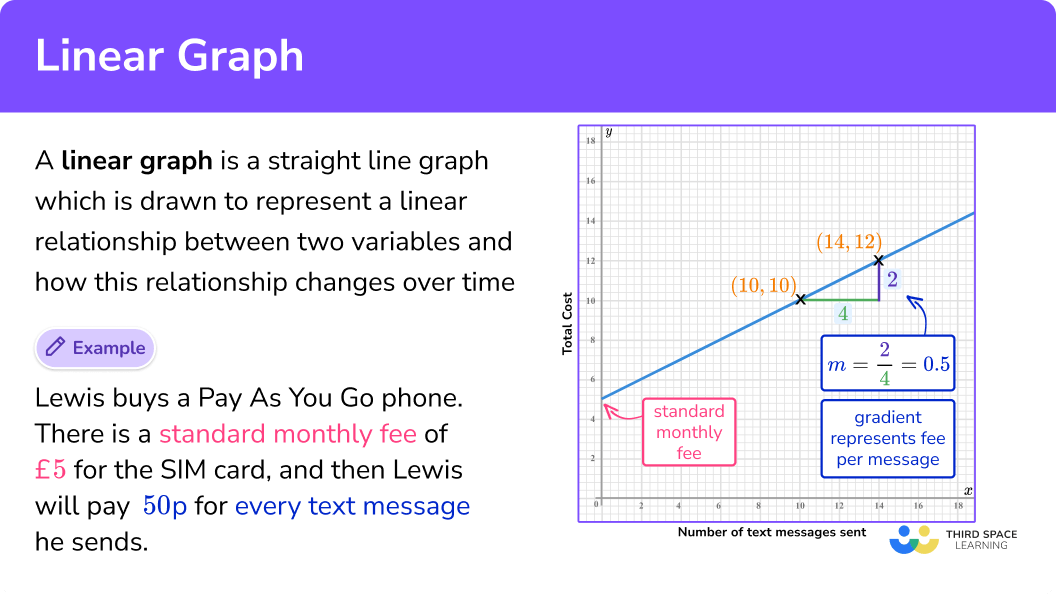### Drawing linear graphs

In order to draw linear graphs (also known as graphs of linear equations) we need to generate pairs of coordinates to plot onto a set of axes.

To generate these we substitute given x values into the equation of the linear graph to work out the corresponding y values. Once we have generated the coordinates, we plot these as a graph.

For example,

y=x+2.

Step-by-step guide: Equation of a line

## How to draw a linear graph

In order to draw a linear graph:

1. Substitute the \textbf{x} coordinate into the equation of a straight line.
2. Plot the paired \textbf{x} and \textbf{y} coordinates onto the axes.
3. Join the points with a straight line.

### Explain how to draw a linear graph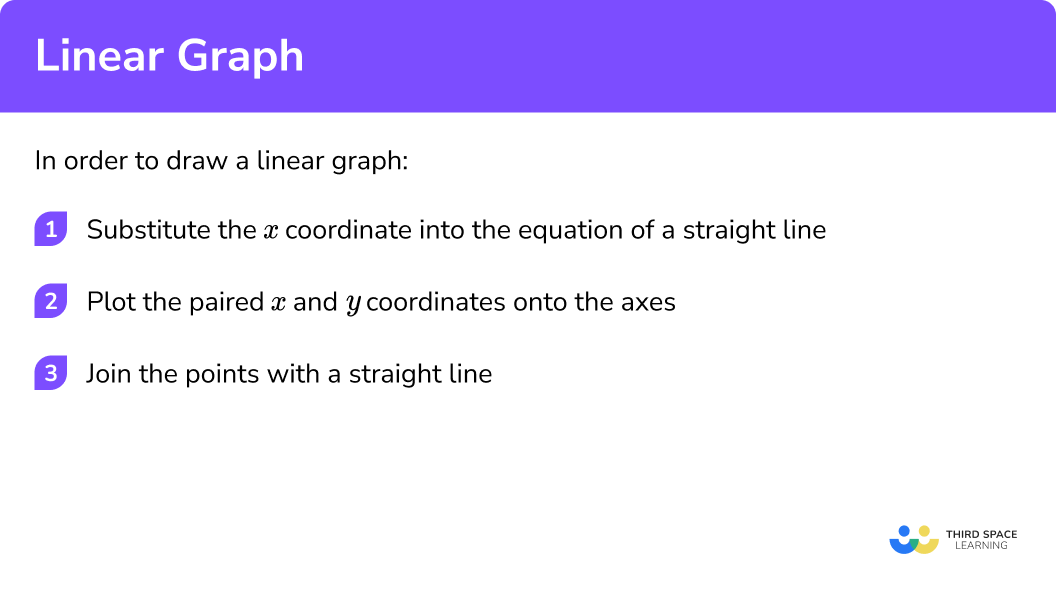### Related lessons on straight line graphs

Linear graph is part of our series of lessons to support revision on straight line graphs. You may find it helpful to start with the main straight line graphs lesson for a summary of what to expect, or use the step by step guides below for further detail on individual topics. Other lessons in this series include:

## Linear graph examples

### Example 1: drawing linear graphs

Draw the graph of the equation y=3x+1 for all values of x from -2 to 2.

1. Substitute the \textbf{x} coordinate into the equation of a straight line.

2Plot the paired \textbf{x} and \textbf{y} coordinates onto the axes.

3Join the points with a straight line.

### Example 2: drawing linear graphs

Draw the graph of the equation y=2x-3 for all values of x from -2 to 2.

Substitute the \textbf{x} coordinate into the equation of a straight line.

Plot the paired \textbf{x} and \textbf{y} coordinates onto the axes.

Join the points with a straight line

### Example 3: drawing linear graphs

Draw the graph of the equation y=5-x for all values of x from -2 to 2.

Substitute the \textbf{x} coordinate into the equation of a straight line.

Plot the paired \textbf{x} and \textbf{y} coordinates onto the axes.

Join the points with a straight line

## Gradient of a linear graph

The gradient of a linear graph tells us how steep the line is. The higher the gradient, the steeper the line. If the gradient is positive, the line goes up, from left to right. If the gradient is negative, the line goes down, from left to right.

For example, the linear graph below has a positive gradient. It moves in an upward direction, from left to right.

This linear graph has a negative gradient. It moves in a downwards direction, from left to right.

Step-by-step guide: Gradient of a line

## How to find the gradient from a linear graph

In order to find the gradient from a linear graph:

1. Select two points on the line that occur on the corners of two grid squares.
2. Sketch a right angle triangle and label the change in \textbf{y} and the change in \textbf{x}.
3. Divide the change in \textbf{y} by the change in \textbf{x} to find \textbf{m}.

### Explain how to find the gradient from a linear graph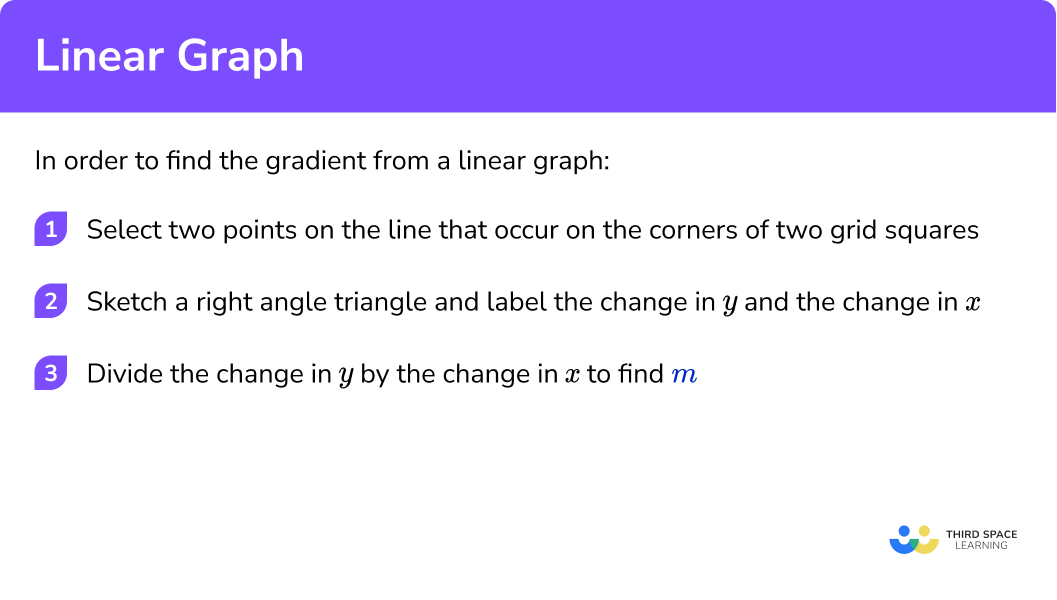## Gradient of a linear graph examples

### Example 4: gradient of a positive linear graph

Find the gradient of the linear graph below.

Select two points on the line that occur on the corners of two grid squares.

Sketch a right angle triangle and label the change in \textbf{y} and the change in \textbf{x}.

Divide the change in \textbf{y} by the change in \textbf{x} to find \textbf{m}.

### Example 5: gradient of a negative linear graph

Find the gradient of the linear graph below.

Select two points on the line that occur on the corners of two grid squares.

Sketch a right angle triangle and label the change in \textbf{y} and the change in \textbf{x}.

Divide the change in \textbf{y} by the change in \textbf{x} to find \textbf{m}.

## Equation of a linear graph

A linear graph is a visual representation of a linear function. All linear graphs can be written as linear equations. These equations take the general form

y=mx+c.

Where m is the gradient of the line and c is the \textbf{y} -intercept.

For example,

y=3x+2.

Here we can see that the gradient is 2, and the y -intercept is at +2.

The graph of this equation would look like this,

Step-by-step guide: Equation of a line

## How to find the equation of a linear graph

In order to find the equation of a linear graph:

1. Calculate the gradient of the line.
2. State the \textbf{y} -intercept of the straight line.
3. Write the equation of the line in the form \bf{y=mx+c.}

### Explain how to find the equation of a linear graph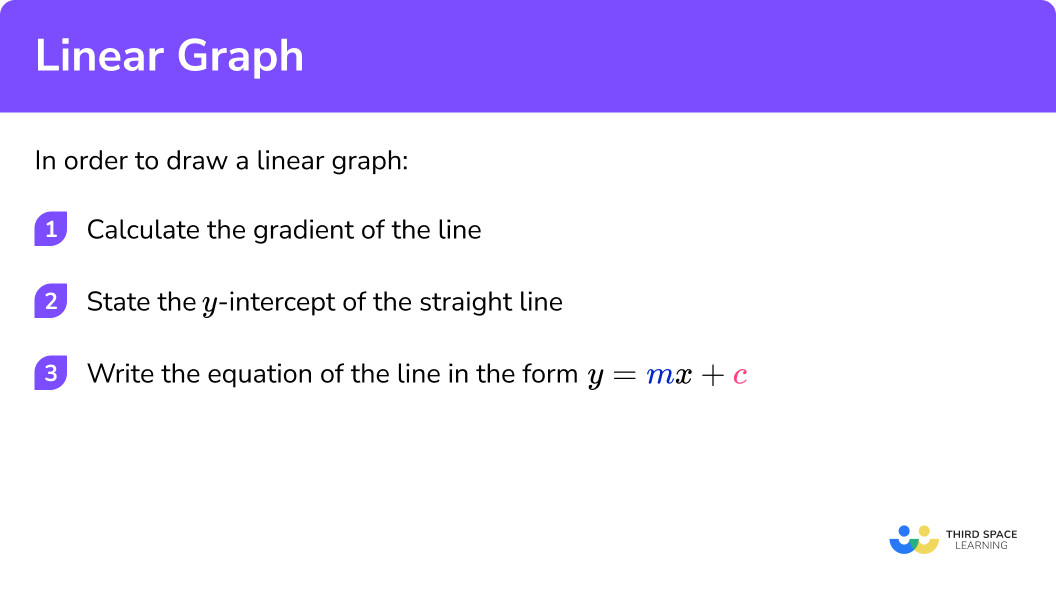## Finding the equation of a linear graph examples

### Example 6: finding the equation of a linear graph

Write the equation of the linear graph below in the form y=mx+c.

Calculate the gradient of the line.

State the \textbf{y} -intercept of the straight line.

Write the equation of the line in the form \bf{y=mx+c.}

## Parallel and perpendicular lines

Parallel lines are lines that travel in exactly the same direction, remaining equidistant from each other, therefore never meet. The gradients of parallel lines are equal.

For example,

Perpendicular lines are lines that intersect at 90 \degree to each other. The gradients of perpendicular lines are the negative reciprocals of each other. In other words, they multiply together and give a product of -1.

For example,

Step-by-step guide: Parallel and perpendicular lines

## How to identify lines parallel or perpendicular to a linear graph

In order to identify lines that are parallel or perpendicular to a linear graph:

1. Calculate the gradient of both lines.
3. State whether the lines are parallel, perpendicular or neither.

### Explain how to identify lines parallel or perpendicular to a linear graph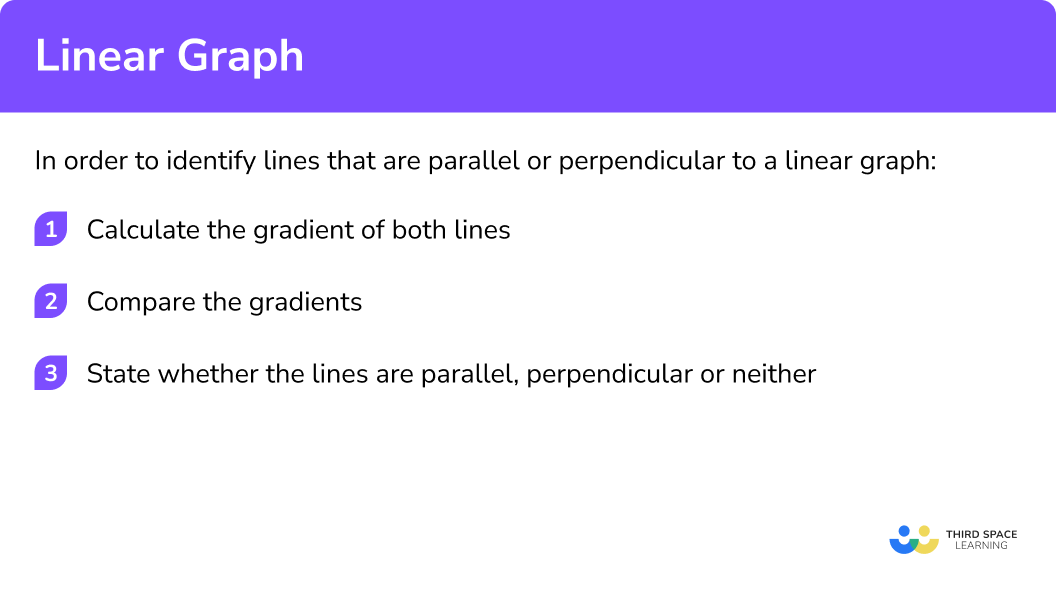## Identifying parallel and perpendicular examples

### Example 7: identifying parallel lines

Show that the linear graphs below are parallel.

Calculate the gradient of both lines.

State whether the lines are parallel, perpendicular or neither.

### Example 8: identifying perpendicular lines

Show that the linear graphs below are perpendicular.

Calculate the gradient of both lines.

State whether the lines are parallel, perpendicular or neither.

### Common misconceptions

• Confusing \textbf{x} and \textbf{y} coordinates

When plotting coordinates, they are in the form (x, \ y).

For example, the coordinate (4, \ 3) would be plotted at the point where 4 on the x -axis and 3 on the y -axis intersect.

• Recognising algebraic notation

When substituting values to generate the coordinates for plotting linear graphs, remember 3x = 3 \times x. Inversely, \frac{x}{3}=x \div 3.

### Practice linear graph questions

1. Complete the table of values to generate the coordinates for a linear graph with the equation y=4x+2.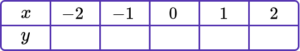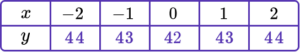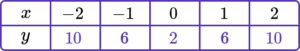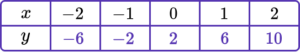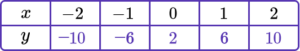y=4x+2 is the equation of the linear graph.

Substituting the x values into the equation, generates the corresponding y values.

\begin{aligned} &y=(4 \times -2)+2=-6 \\\\ &y=(4 \times -1)+2=-2 \\\\ &y=(4 \times 0)+2=2 \\\\ &y=(4 \times 1)+2=6 \\\\ &y=(4 \times 2)+2=10 \end{aligned}

2. Complete the table of values to generate the coordinates for a linear graph with the equation y=2x-1.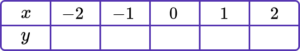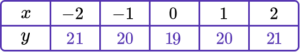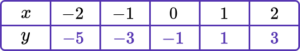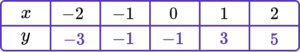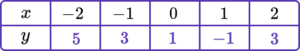y=2x-1 is the equation of the linear graph.

Substituting the x values into the equation, generates the corresponding y values.

\begin{aligned} &y=(2 \times -2)-1=-5\\\\ &y=(2 \times -1)-1=-3 \\\\ &y=(2 \times 0)-1=-1 \\\\ &y=(2 \times 1)-1=1 \\\\ &y=(2 \times 2)-1=3 \end{aligned}

3. Find the gradient of the linear graph below.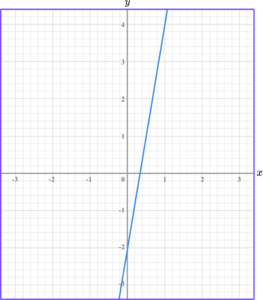\frac{1}{6}4\frac{1}{4}6The change in y is y_2-y_1=4- -2=6.

The change in x is x_2-x_1=1-0=1.

The formula for the gradient of a linear graph is

So, we do the change in y \ \div change in x.

For this linear graph, the gradient is 6 \div 1=6.

4. Write the equation of the linear graph below in the form y=mx+c.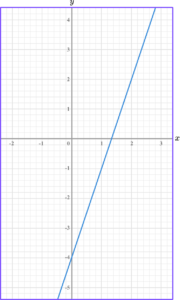y=3x-4y=-3x-4y=4x-3y=\frac{1}{3}x-4The y -intercept is -4.

The equation of the linear graph is y=3x-4.

5. Write the equation of the linear graph below in the form y=mx+c.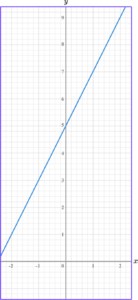y=5x-2y=2x+5y=2x-5y=5x+2The y -intercept is 5.

The equation of the linear graph is y=2x+5.

6. Which equation of a linear graph is parallel to y=6x-1?

y=6x+10y=x+6y=x-1y=2x-1Parallel lines have the same gradient. The gradient of the linear graph y=6x-1 is 6, so the equation which is parallel from the line is y=6x+10.

### Linear graph GCSE questions

1. (a) Complete the table of values for y=2x+5.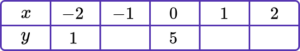(b) On the grid, draw the graph of y=2x+5 for values of x from -2 to 2.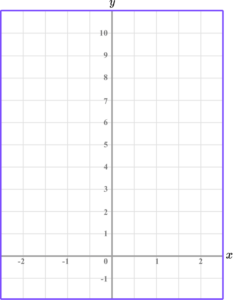(4 marks)

(a)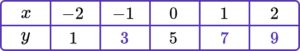1 correct value.

(1)

All 3 correct values.

(1)

(b)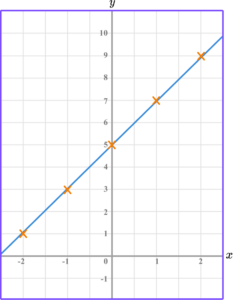All coordinates plotted correctly.

(1)

Straight line drawn connecting all coordinates.

(1)

2. Write the equation of the linear graph below in the form y=mx+c.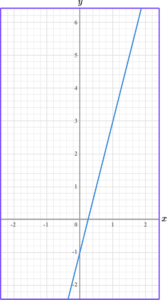(3 marks)

(1)

y -intercept identified as -1.

(1)

y=4x-1

(1)

3. Write down the equation of a line parallel to y=3x+2.

(1 mark)

Any equation written in the form y=mx+c starting y=3x.

(1)

## Learning checklist

You have now learned how to:

• Plot linear graphs
• Identify the gradient of a linear graph
• Find the equation of a linear graph
• Identify parallel and perpendicular lines

## Still stuck?

Prepare your KS4 students for maths GCSEs success with Third Space Learning. Weekly online one to one GCSE maths revision lessons delivered by expert maths tutors.

Find out more about our GCSE maths tuition programme.